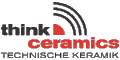Breviary Technical Ceramics

 Properties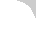BreviaryContentIntroductionHistoryMaterialsManufacturingProperties-General-Overview-Mechanical·Density·Porosity·StrengthBendingCompressionTensileWeibullKIC ·Elasticity·Hardness-Thermal-Electrical-Corrosion-WearApplicationsDesignJoiningQualityAppendixBibliographyRegister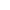Breviary OrderformImprintdata privacy#### 5.3.3.4 Weibull modulus

Using a theory developed by Weibull, based on the concept of the failure of the weakest link, the strength distribution of ceramic materials can be described effectively in mathematical terms. An important assumption is that the failure is caused by a single "failure type" (structural inhomogeneity). Weibull chose to describe the strength behaviour using a special form of extreme value distribution; this was later named the Weibull distribution in his honour.
This results in a definite relationship between mechanical load and the failure probability of a part if the distribution parameters are known. Using a level of strength at which the failure probability becomes 63.2 % (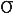0), the Weibull modulus (m) becomes a measure of the distribution of strengths. The higher the Weibull modulus is, the more consistent the material (which means that uniform "defects" are evenly distributed throughout the entire volume) and also the narrower the probability curve of the strength distribution. Today, values between 10 < m < 20 are typically achieved. These values are determined for ceramic materials usually using the four-point bending method, since measuring the tensile strength of these materials is extremely difficult.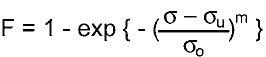F   = failure probability= stressu = minimum strength m = Weibull modulus0 = Location parameter of the Weibull distribution, strength at 63.2% failure probability; in the Weibull distribution this plays the role of the mean value in a normal distribution. Typically,u = 0 is used

Since the number of possible defects in this ceramic is dependent on the volume of the part, the volume under load must be taken into account. The strength of larger parts is thus less than what is measured on test samples. However, with large volumes under load, a larger Weibull modulus results in higher load capacity. Using Weibull statistics, the following component strength results from the volume relationship: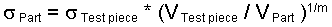The volume under stress is to be inserted here for the component volume, and the stress distribution must be identical (e.g.tensile or4pb). This relationship does not apply to compressive stress!

Test procedures for determining the Weibull modulus are specified in DIN EN 843-5 and DIN 51 110-3.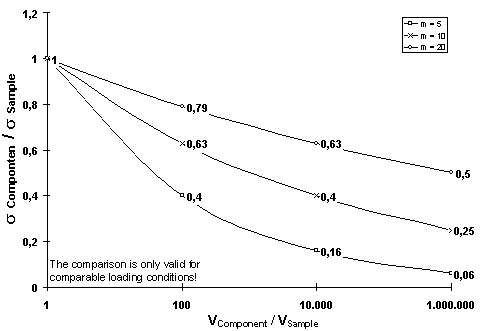Figure 85: Relationship between component size and strength for
different values of Weibull modulus, m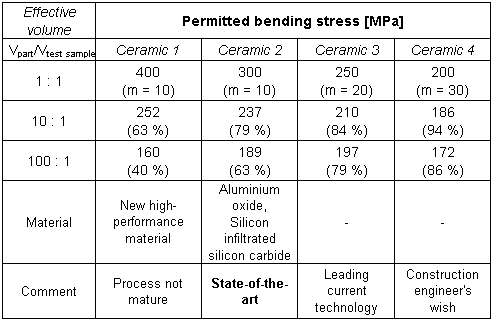Table 10:
Comparison of the strengths of components and test pieces for
different strength scatters (Weibull modulus, m)info@keramverband.de